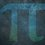# Erdős–Moser Equation Proof (Part $2$ - The Facts)

In Part $1$ of this series, I said (in detail) that we are going to attempt to prove that

$1^k + 2^k + ... + m^k = (m + 1)^k$

has more than $1$ solution using the equation

$m^k - (m + 1)^k = - 1 - 2^k$

as

$1^k = 1$

Now, we need to lay down the foundations.

Using the first constraint,

$k = 1, 2$

as $x$ needs to be whole.

The second and fifth constraints would contradict the final constraint, hence no need for them.

The first half of the third constraint is useful - using OEIS, the LCM of the first $200$ natural numbers is

$337293588832926264639465766794841407432394382785157234228847021917234018060677390066992000$

The second half of the third constraint is to be used in the proof.

The fourth constraint is obsolete due to the final constraint.

As for the final constraint, we know that

$m > 2.73129 \times 10^{1,667,658,416}$

But what's the value of $k$?

Going into an AMS paper referenced by the Wikipedia page of the Erdős–Moser equation (here is the paper: Erdős–Moser Equation Final Constraint Computations and Proofs Paper),

$k \geq 2$

therefore

$k = 2$

We've got our values of $m, k$.

We're ready to do some algebraic proof!Note by Yajat Shamji
5 months, 2 weeks ago

This discussion board is a place to discuss our Daily Challenges and the math and science related to those challenges. Explanations are more than just a solution — they should explain the steps and thinking strategies that you used to obtain the solution. Comments should further the discussion of math and science.

When posting on Brilliant:

• Use the emojis to react to an explanation, whether you're congratulating a job well done , or just really confused .
• Ask specific questions about the challenge or the steps in somebody's explanation. Well-posed questions can add a lot to the discussion, but posting "I don't understand!" doesn't help anyone.
• Try to contribute something new to the discussion, whether it is an extension, generalization or other idea related to the challenge.

MarkdownAppears as
*italics* or _italics_ italics
**bold** or __bold__ bold
- bulleted- list
• bulleted
• list
1. numbered2. list
1. numbered
2. list
Note: you must add a full line of space before and after lists for them to show up correctly
paragraph 1paragraph 2

paragraph 1

paragraph 2

[example link](https://brilliant.org)example link
> This is a quote
This is a quote
    # I indented these lines
# 4 spaces, and now they show
# up as a code block.

print "hello world"
# I indented these lines
# 4 spaces, and now they show
# up as a code block.

print "hello world"
MathAppears as
Remember to wrap math in $$ ... $$ or $ ... $ to ensure proper formatting.
2 \times 3 $2 \times 3$
2^{34} $2^{34}$
a_{i-1} $a_{i-1}$
\frac{2}{3} $\frac{2}{3}$
\sqrt{2} $\sqrt{2}$
\sum_{i=1}^3 $\sum_{i=1}^3$
\sin \theta $\sin \theta$
\boxed{123} $\boxed{123}$